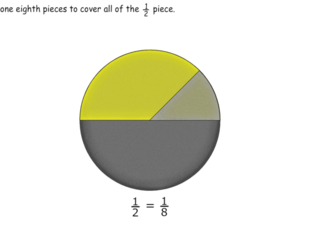# Interactive Problem Bank

•Fraction Circles
•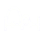Number Lines
•Fraction Bars
•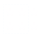Sets
•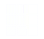Area Models
•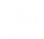Base Ten Blocks

## Making Sense of Fractions

• Students use visual models to name fractions as a⁄b where a is how many parts are represented and b is how many equal-sized parts are in the whole. The whole is equal to whole fraction circles, other fractions, and sets of objects.
• Students determine if two fractions are equivalent if they are the same size.

### Part of a Whole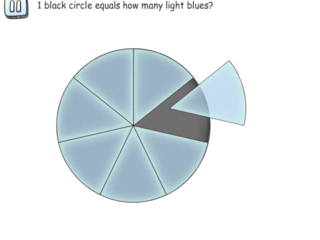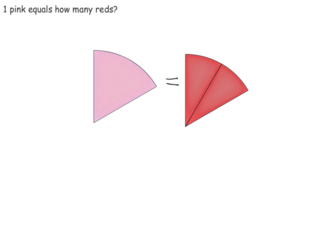### Fraction Word Names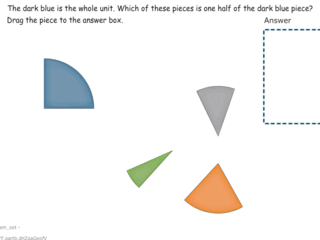### Connecting Model and Symbol - Bars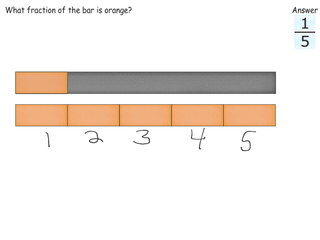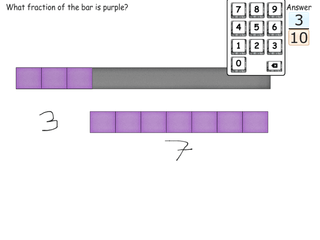### Connecting Model and Symbol - Mixed Shapes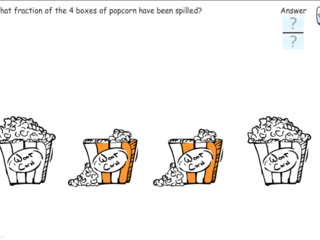### Modeling Fractions with Bars### Modeling Fractions with Sets of Objects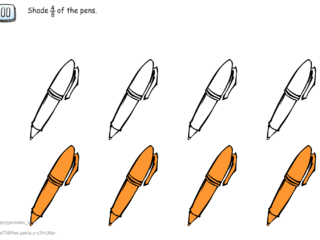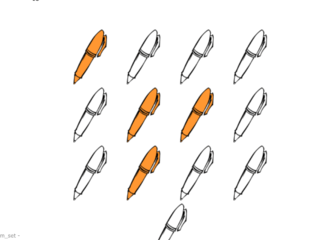### Modeling Fractions with Circles### Relating Fraction Pieces to Each Other